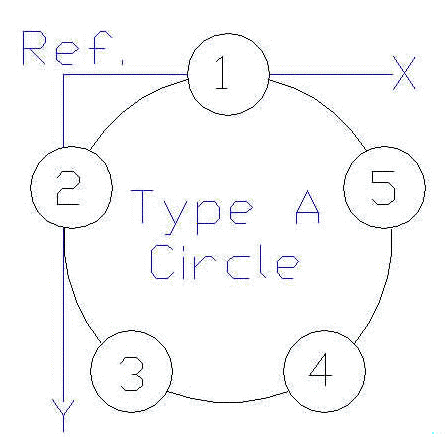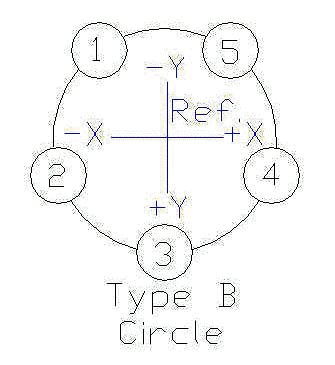# Bolt Hole Circles

Many of us need to bore holes in a circle but don’t have a rotary table, don’t want to remove the vise or need to create a circle too big for the table. There is a solution in Machinery’s Handbook. Find the section on JIG BORING in your copy of the Handbook. In my 23rd edition, it starts on page 950. Look for the four tables labeled “Hole Coordinate Dimension Factors for Jig Boring.” Definitions: There are two types of bolt hole circles:
1. Those that start with hole number 1 centered at the top of the circle are a Type A circle
2. Those that start with the holes evenly centered around the top of the circle are a Type B circle.
It is also convenient to have tables for circles with two different reference points: 1. Bolt hole circles where the reference is found by the intersection of two tangential lines drawn vertically at the left of the circle and horizontally at the top of the circle as shown in this first drawing.and 2. Bolt hole circles where the reference is found at the origin or center of the circle as shown in the second drawing below.The two types of hole layouts and the two reference locations create the need for the four tables. As with any system based on coordinates, we have X and Y directions for movement, just as we do on our milling tables. Now for the tables themselves: As you look at Table 1 in your copy of Machinery’s Handbook, you notice the table is broken into groups by the number of holes per circle. You will also notice that there are no numbers larger than 1. That is because these numbers are based on a bolt hole circle with a diameter of 1. So, if you want to make a bolt hole circle with a diameter of 2 (inches, millimeters or furlongs, it doesn’t matter) you just multiply the numbers by 2. The same method works for a circle of any diameter including those with a diameter smaller than 1. Suppose we want to drill three holes on a circle where the first hole is at the top center of the circle and the circle diameter is 4 inches. Furthermore, we are going to use as a reference point for the circle, the center of the circle. We have four important pieces of information to help us select the proper table and create the X-Y coordinate pairs for the three holes:
1. It is a three hole circle.
2. The first hole is at the top center.
3. The reference point is the center of the bolt circle.
4. The diameter of the bolt circle is 4 inches.
Armed with these facts, we can select Table 3 because it deals with bolt hole circles where the reference point is in the center of the circle and where the first hole is at the top center. Next we locate the group of coordinates under the label ‘3 Holes’. To calculate the coordinates for our 4 inch bolt hole circle, all we have to do is multiply each of those coordinate values by the value 4 inches. Getting out our trusty paper and pencil (and maybe a calculator), we multiply each of the three sets of X-Y coordinate values by 4 to arrive at the following table for our 4 inch bolt hole circle:
 Coordinate Name Table 3 Value Bolt Hole Circle Diameter Calculated Coordinate Value X1 +0.00000 x 4 = 0.0 Y1 -0.50000 x 4 = -2.0 X2 -0.43301 x 4 = -1.73 Y2 +0.25000 x 4 = 1.0 X3 +0.43301 x 4 = 1.73 Y3 +0.25000 x 4 = 1.0
Now, locate the center of your workpiece under the spindle of your mill and zero your table position dials or DRO readout. Then move the mill table to the coordinates just calculated and drill/bore your holes. The coordinate pair, X1+Y1 give you the location of hole number one, X2+Y2, the second hole, and X3+Y3 the location of the third hole. Remember that positive numbers are located relative to the circle center as shown in the Type B Circle. The holes will be located within the accuracy of your table position reading device. Author: Mike Rehmus
This div height required for enabling the sticky sidebar